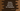# How to calculate gross pay for hourly paid employees in Python## How to calculate gross pay for hourly paid employees in Python:

Gross pay is the total amount paid to an employee for an interval of time. This post will show you how to calculate gross pay for hourly paid employees.

Hourly paid employees are paid hourly, i.e. payment is calculated based on the number of hours the employee worked. The gross pay for an employee is calculated on weekly basis. If we consider 8-hours work rule, the payment will be calculated for 40 hours. Also, employees are paid for overtime, normally more than or equal to 1.5 times normal pay per hour.

### Python program to calculate gross pay for hourly paid employees:

Our program will find the gross pay considering 40 hours a week on hourly payment and for overtime, it will consider 1.5 times normal pay per hour.

It will take the hourly wage and total number of hours to work as inputs from the user, calculate and print the gross pay.

Below is the complete python program:

``````def calculate_gross_pay(hours, wage):
if hours > 40:
extra_hours = hours - 40
total_pay = 40 * wage + extra_hours * 1.5 * wage

return hours * wage

if __name__ == '__main__':
hours = int(input('Enter total number of hours worked: '))
wage = int(input('Enter per hour payment in \$: '))

print('Gross pay: \${}'.format(calculate_gross_pay(hours, wage)))``````

### Explanation:

Here,

• calculate_gross_pay method is used to calculate the gross payment. This method takes the hours value and wage value as parameters and returns the gross pay value.
• This method checks if the hours is more than 40 or not. If it is more than 40, this method calculates the extra hours worked and calculates the gross pay based on that value.
• This program takes the total working hours and per hour payment values as inputs from the user. It calculates the gross pay and prints that value to the user.

### Sample output:

It will print output as like below:

``````Enter total number of hours worked: 20
Enter per hour payment in \$: 200
Gross pay: \$4000

Enter total number of hours worked: 50
Enter per hour payment in \$: 30
Gross pay: \$1650.0``````